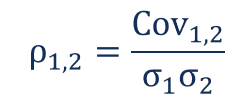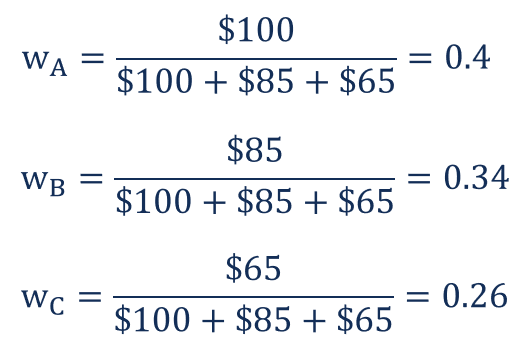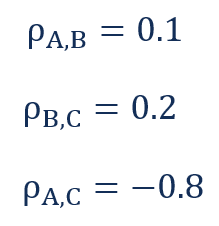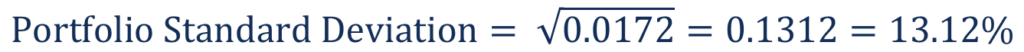# Portfolio Variance

A statistical value that assesses the degree of dispersion of a portfolio's returns

## What is Portfolio Variance?

Portfolio variance is a statistical value that assesses the degree of dispersion of the returns of a portfolio. It is an important concept in modern investment theory. Although the statistical measure by itself may not provide significant insights, we can calculate the standard deviation of the portfolio using portfolio variance.The calculation of portfolio variance considers not only the riskiness of individual assets but also the correlation between each pair of assets in the portfolio. Thus, the statistical variance analyzes how assets within a portfolio tend to move together. The general rule of portfolio diversification is the selection of assets with a low or negative correlation between each other.

CFI’s Math for Corporate Finance Course explores the financial mathematics concepts required for Financial Modeling.

### Formula for Portfolio Variance

The variance for a portfolio consisting of two assets is calculated using the following formula:Where:

• wi – the weight of the ith asset
• σi2 – the variance of the ith asset
• Cov1,2 – the covariance between assets 1 and 2

Note that covariance and correlation are mathematically related. The relationship is expressed in the following way:Where:

• ρ1,2 – the correlation between assets 1 and 2
• Cov1,2 – the covariance between assets 1 and 2
• σ1 – the standard deviation of asset 1
• σ2 – the standard deviation of asset 2

Knowing the relationship between covariance and correlation, we can rewrite the formula for the portfolio variance in the following way:The standard deviation of the portfolio variance can be calculated as the square root of the portfolio variance:Note that for the calculation of the variance for a portfolio that consists of multiple assets, you should calculate the factor 2wiwjCovi.j (or 2wiwjρi,j,σiσj) for each possible pair of assets in the portfolio.

### Example of Portfolio Variance

Fred holds an investment portfolio that consists of three stocks: stock A, stock B, and stock C. Note that Fred owns only one share of each stock. Information about each stock is given in the table below:Fred wants to assess the risk of the portfolio using portfolio variance and portfolio standard deviation.

First, he needs to determine the weights of each stock in the portfolio. This can be done by dividing the total value of each stock by the total portfolio value.In addition, he needs to know the correlation between each pair of stocks. His calculations show the following correlations:The portfolio variance can then be calculated in the following way:CFI offers the Financial Modeling & Valuation Analyst (FMVA)™ certification program for those looking to take their careers to the next level. To keep learning and advancing your career, the following resources will be helpful:

• Financial Modeling Courses
• Correlation
• Negative Correlation
• Regression Analysis

### Financial Analyst Training

Get world-class financial training with CFI’s online certified financial analyst training program!

Gain the confidence you need to move up the ladder in a high powered corporate finance career path.

Learn financial modeling and valuation in Excel the easy way, with step-by-step training.